# “波束成形” 是个什么玩意

## 未来无线通信的背景

5G/+5G 主要引入了 Massive MIMO，以及毫米波技术。

• MIMO 技术特点是可以在基站端配备几根或者数十根天线阵元，并且这些天线在发送信号时都是相互独立的，同时从发射端传来的信号将会被接收端多根天线阵元接收，然后通过其他技术恢复出有效的原始信号。Massive MIMO 技术则通过增加基站端的天线数量，扩展系统空间自由度，降低噪声、小区内干扰等。Massive MIMO 系统的信号处理方法不需要再采用复杂的非线性设计来避免干扰，而只需要简单的线性设计即可实现较好的系统性能。

• 对于 Massive MIMO 系统来说，提高天线增益对于获取较为精准的信道状态信息有着不可忽视的重要性，当 Massive MIMO 系统在基站端配置大量天线的时候，不仅提高系统硬件的复杂度，还加大了成本消耗，对于这点学术工作者主要结合毫米波特性，可以采用均匀天线阵列，形成波束信号来降低使用基站端射频（RF）链路，同时还可以增大天线的增益。
但 Massive MIMO 系统在基站端增加天线数量之后，获取信道状态信息就不是那么容易，考虑到下行链路导频资源的消耗问题和毫米波的衰落问题，在研究 Massive MIMO 系统的时候通常采用 TDD 系统模型

### a. 大规模 MIMO

Massive MIMO 系统为了获得更大的增益在发射端可以布置庞大数量的天线。在 Massive MIMO 系统中，随着天线数量增加可以屏蔽非相关噪声的影响与小尺度衰落，并且每个天线阵元的发送功率也会随之变小。

#### 大规模 MIMO 系统相比传统 MIMO 的优点：

• 减轻衰落：Massive MIMO 系统中天线数量多达上百根，信号陷入小尺度衰落的概率比较小，小尺度衰落的影响可能被屏蔽掉，于此系统性能将只与大尺度衰落紧密相关。
• 降低了系统成本、功耗和复杂度：Massive MIMO 有大量剩余自由度，降低对放大器和射频链路的精确度和线性度的要求。Massive MIMO 系统采用小型有源天线单元，成本比传统 MIMO 系统要低。此外，Massive MIMO 可以采用简单的线性处理，如最大比发送或者接收就可以实现系统的最优性能，简化了系统的复杂度。
• 降低干扰：Massive MIMO 随着基站安装的天线数目累加增多，传播的信号导频逐渐呈现正交性，小区内用户之间干扰就会大大降低同时可在基站侧使用波束成形技术，将大量天线的能量集中于较小的空间域，形成比较窄的小范围内的信号波束对准目标用户，增强信号在空间的正交性，抑制干扰。

#### Massive MIMO 系统的不足：

• 信道估计准确性：参考信号开销、信道的变化速度、覆盖环境的复杂度、信道估计精确度、反馈信息的时延以及反馈开销、计算量都是影响 CSI 准确性的关键因素。
• 计算复杂度：信道估计算法随着天线数量的不断增加，人们对其优化要求也越来越高，导致计算复杂度加大 。
• 导频污染：Massive MIMO 在 TDD 模式下存在导频污染问题。系统连接用户增多，增加导频的负载，提升导频污染，因而寻求如何更有效的降低导频污染的算法也是刻不容缓。

### b. 毫米波

• 数字基带预编码 DBF
考虑接收机端，数字基带预编码是在模数转换（ADC）之后用矩阵处理调制的符号流。该方案要求 RF 链数量和天线数目相同，能达到很好的系统性能。在数字基带预编码中，传统的线性和非线性预编码都可以直接应用到大规模 MIMO 系统中，但非线性预编码的计算复杂度过高，线性方案更占优势。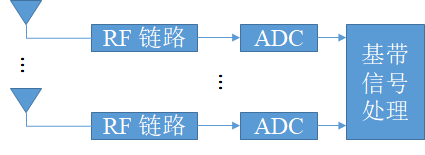• 模拟射频预编码 ABF
考虑接收机端，模拟预编码是在模数转换（ADC）之前对输入符号流进行处理。该技术在毫米波大规模 MIMO 系统的收发两端采用移相器网络将天线阵列与一个 RF 链相连，从而能够执行模拟预处理。虽然该技术在一定程度上降低了系统的能量消耗和成本，但其只支持单数据流传输，且不能提供空间多路复用增益。这类方案可将多根天线同时连到一条 RF 链上，非常适用于大规模 MIMO 系统天线数很多的情况，能显著降低系统硬件成本，且计算复杂度较低。模拟预编码能显著减少系统硬件开销，但需要牺牲部分性能。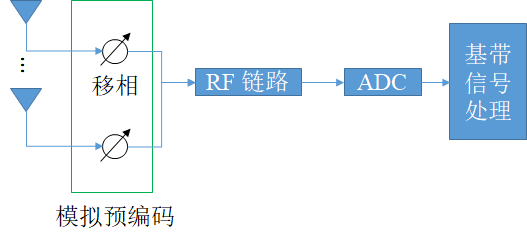• 混合预编码
混合预编码作为近年来兴起的方案，能结合数字预编码和模拟预编码的优点，在硬件开销和系统性能之间折中。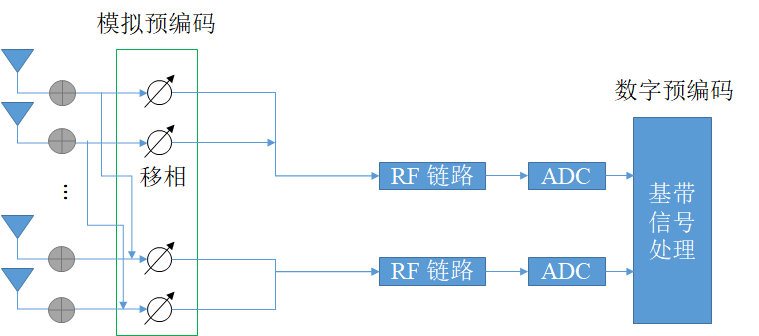图中都忽略了毫米波功率放大器（PA）与低噪放大器（LNA）。

### c. 预编码 = 波束成形 ？

precoding 和 beamforming 不相同！他们属于不同的技术领域，但现有不少文献总把 precoding 和 beamforming 等同。

• beamforming 是阵列信号处理领域的。
• precoding 是通信系统里解决 ISI 问题的，是纯正的通信问题。

## 毫米波 Massive MIMO 系统中的 波束成形

$\mathbf x =\left[x_1,x_2,\cdots,x_N\right]^T = \mathbf A(\theta) x_1$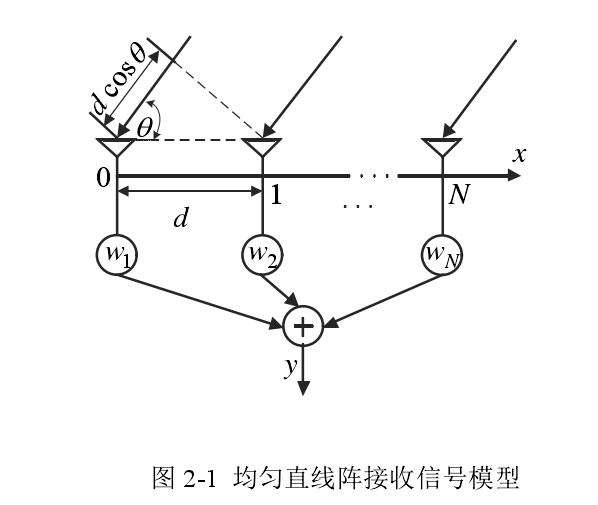$y =\mathbf w^T \mathbf x+ n$

### 一个例子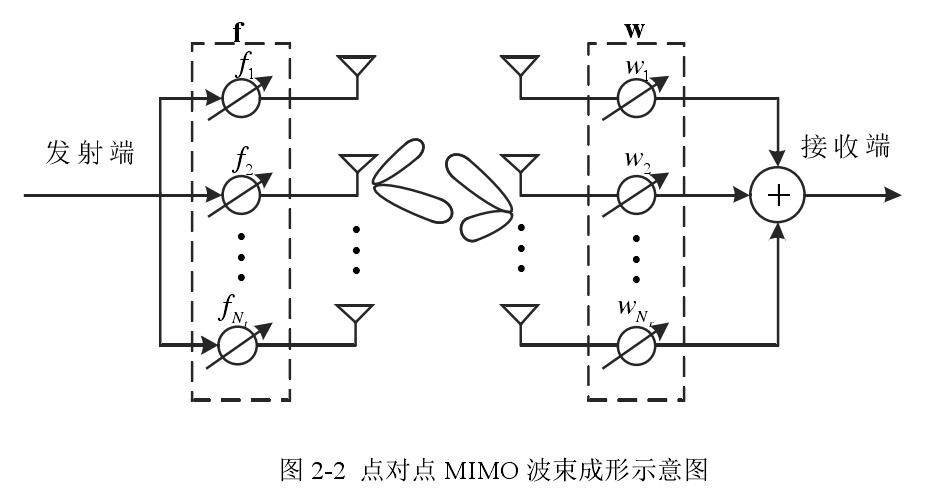$\mathbf {y = HFx+n}$

$\mathbf W^H \mathbf y = \mathbf W^H\mathbf {HFx}+ \mathbf W^H \mathbf n$

$\mathbf H = \mathbf{U \Sigma V}^H$

• $\mathbf{\Sigma }_{n\times m}$ 的对角线为非负实数，称为奇异值，从大到小排列。
• $\mathbf{UU}^H=\mathbf{U}^H\mathbf{U} = \mathbf I_{n}$
• $\mathbf{VV}^H=\mathbf{V}^H\mathbf{V} = \mathbf I_{m}$

${\mathbf y = \mathbf{U\Sigma V^H Vx}+\mathbf n}=\mathbf {U\Sigma x+n}$

$\mathbf {U^H y = U^H U \Sigma x + U^H n} \\ \mathbf{y^* = \Sigma x + n}^*$

### 从预编码到波束成形

$\mathbf {y_{n\times 1} = H_{n\times m}F_{m \times r}x_{r\times 1}+n_{n\times 1}}$

$\mathbf F_t=\underset{\mathbf F}{\text{argmax}} \left[ (\mathbf{HF})^H \mathbf {HF}\right]$
$\mathbf F$ 的理想选择是 SVD 中的 $\mathbf V$ 矩阵里面最大的前 $r$ 个奇异值对应的列组成的矩阵。同样，在接收端，接收波束成形矩阵 $\mathbf W_r$ 的列由 $\mathbf U$$r$ 个最大的奇异值对应的列构成。

$\begin{bmatrix}1\\ 0\end{bmatrix}$

$\mathbf {y = H F x+n}= \frac{1}{\sqrt{M}} \mathbf {a^T(\theta)a^*(\theta) x +n}=\sqrt{M}\mathbf {x+n}$